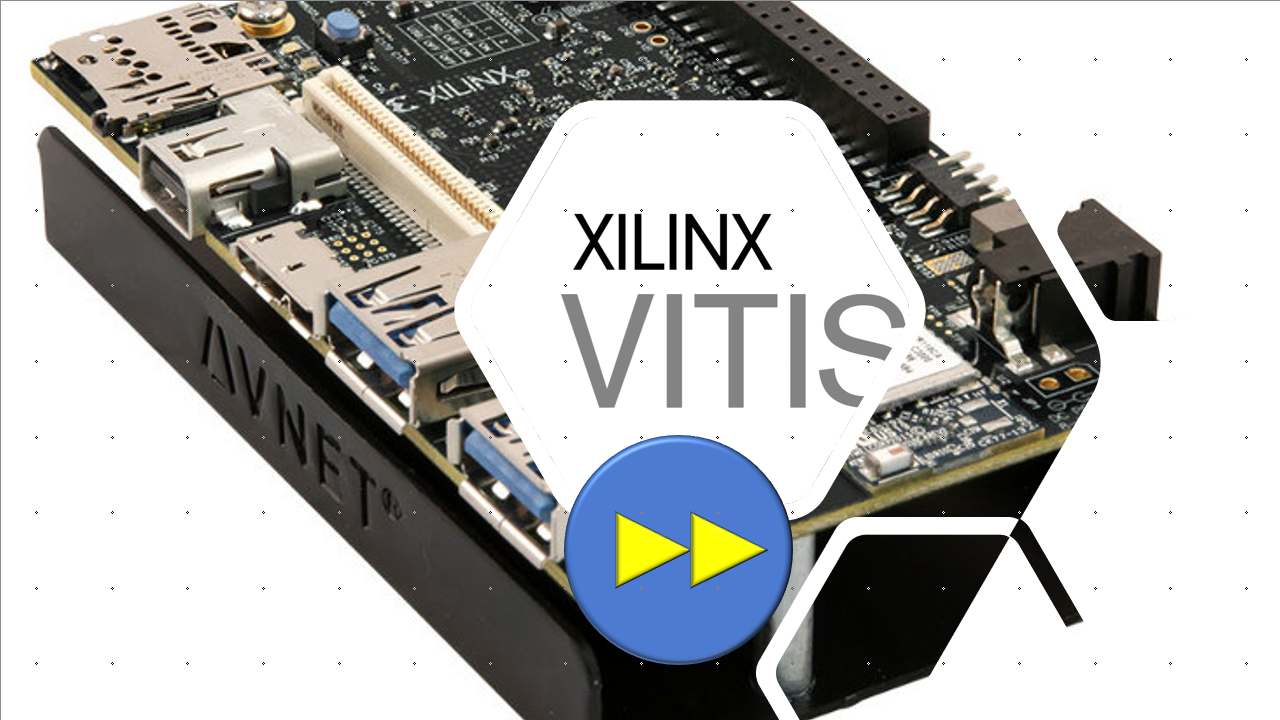Wed. Dec 6th, 2023

# Embedded Hardware Accelerator with Xilinx Vitis: Part 4: Bandwidth OptimisationIn the previous blog, I explained how to implement the image thresholding example in Vitis for ZynqMPSOC. Here, I am going to optimise the kernel and reduce the execution time and make the hardware about 13x faster. If you would like to learn how to code hardware in HLS please refer here.

In the previous implementation, the image thresholding kernel reads the image pixel-by-pixel, which means 8-bit at each time via HP0 port.

But we know that HP0 port supports 128-bit databus. So why not utilise all the bus bit-width. For this purpose, the kernel should read 16 pixels at a time and process them concurrently.

For this purpose, we can modify the code as follows:

```void read_data(
ap_uint<BUS_WIDTH>   *input_image,
hls::stream<unsigned char> input_pixel_fifo[NUMBER_OF_BYTES],
unsigned int  n,
unsigned int  m )
{
ap_uint<BUS_WIDTH> input_pixels;

for (int i = 0; i < (n*m)/NUMBER_OF_BYTES; i++) {
#pragma HLS PIPELINE
input_pixels = input_image[i];
for (int j = 0; j < NUMBER_OF_BYTES; j++) {
input_pixel_fifo[j] << input_pixels((j+1)*8-1, j*8);
}
}
}

void thresholding(
hls::stream<unsigned char> input_pixel_fifo[NUMBER_OF_BYTES],
hls::stream<unsigned char> output_pixel_fifo[NUMBER_OF_BYTES],
unsigned int  n,
unsigned int  m,
unsigned int  type,
unsigned int  threshold,
unsigned int  maxVal ) {

for (unsigned int i = 0; i < n*m/NUMBER_OF_BYTES; i++) {
#pragma HLS PIPELINE
for (int j = 0;j < NUMBER_OF_BYTES; j++) {
unsigned char output_pixel;
output_pixel = (input_pixel > threshold)? maxVal : 0;
output_pixel_fifo[j] << output_pixel;
}
}
}
void write_data(
hls::stream<unsigned char> output_pixel_fifo[NUMBER_OF_BYTES],
ap_uint<BUS_WIDTH>   *output_image,
unsigned int  n,
unsigned int  m )
{
ap_uint<BUS_WIDTH> output_pixels;

for (int i = 0; i < n*m/NUMBER_OF_BYTES; i++) {
#pragma HLS PIPELINE
for (int j = 0; j < NUMBER_OF_BYTES; j++) {
}
output_image[i] = output_pixels;
}
}

extern "C" {
void image_thresholding (
ap_uint<BUS_WIDTH>   input_image[N_MAX*M_MAX],
ap_uint<BUS_WIDTH>   output_image[N_MAX*M_MAX],
unsigned int  n,
unsigned int  m,
unsigned int  type,
unsigned int  threshold,
unsigned int  maxVal
) {

#pragma HLS DATAFLOW
hls::stream<unsigned char> input_pixel_fifo[NUMBER_OF_BYTES];
hls::stream<unsigned char> output_pixel_fifo[NUMBER_OF_BYTES];

thresholding(input_pixel_fifo, output_pixel_fifo, n, m, type, threshold, maxVal);
write_data(output_pixel_fifo, output_image, n, m);

}
}
```

Now, if we synthesis this kernel and run that on the Ultra96v2 ZynqMPSoC board, the execution time would be 0.45600 ms, which is 13.375 times faster than the previous implementation.

Still, there are lots of room for performance improvement. One way is utilising all the HP ports available between the Zynq MPSOC and DDR memory. We know that there are four HP ports and two HPC posts, each of which supports a 128-bit data bus.